# The REG Procedure

### Multivariate Tests

The MTEST statement described in the section MTEST Statement can test hypotheses involving several dependent variables in the form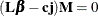whereis a linear function on the regressor side,
is a matrix of parameters,is a column vector of constants,is a row vector of ones, and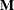is a linear function on the dependent side. The special case where the constants are zero is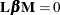To test this hypothesis, PROC REG constructs two matrices calledand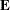that correspond to the numerator and denominator of a univariate F test: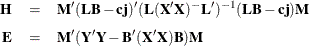These matrices are displayed for each MTEST statement if the PRINT option is specified.

Four test statistics based on the eigenvalues of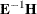or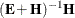are formed. These are Wilks’ lambda, Pillai’s trace, the Hotelling-Lawley trace, and Roy’s greatest root. These test statistics are discussed in Chapter 4: Introduction to Regression Procedures.

The following code creates MANOVA data from Morrison (1976):

* Manova Data from Morrison (1976, 190);
data a;
input sex $drug$ @;
do rep=1 to 4;
input y1 y2 @;
sexcode=(sex='m')-(sex='f');
drug1=(drug='a')-(drug='c');
drug2=(drug='b')-(drug='c');
sexdrug1=sexcode*drug1;
sexdrug2=sexcode*drug2;
output;
end;
datalines;
m a  5  6  5  4  9  9  7  6
m b  7  6  7  7  9 12  6  8
m c 21 15 14 11 17 12 12 10
f a  7 10  6  6  9  7  8 10
f b 10 13  8  7  7  6  6  9
f c 16 12 14  9 14  8 10  5
;


The following statements perform a multivariate analysis of variance and produce Figure 97.49 through Figure 97.52:

proc reg;
model y1 y2=sexcode drug1 drug2 sexdrug1 sexdrug2;
y1y2drug: mtest y1=y2, drug1,drug2;
drugshow: mtest drug1, drug2 / print canprint;
run;


Figure 97.49: Multivariate Analysis of Variance: REG Procedure

The REG Procedure
Model: MODEL1
Dependent Variable: y1

Analysis of Variance
Source DF Sum of
Squares
Mean
Square
F Value Pr > F
Model 5 316.00000 63.20000 12.04 <.0001
Error 18 94.50000 5.25000
Corrected Total 23 410.50000

 Root MSE R-Square 2.29129 0.7698 9.75 0.7058 23.5004

Parameter Estimates
Variable DF Parameter
Estimate
Standard
Error
t Value Pr > |t|
Intercept 1 9.75000 0.46771 20.85 <.0001
sexcode 1 0.16667 0.46771 0.36 0.7257
drug1 1 -2.75000 0.66144 -4.16 0.0006
drug2 1 -2.25000 0.66144 -3.40 0.0032
sexdrug1 1 -0.66667 0.66144 -1.01 0.3269
sexdrug2 1 -0.41667 0.66144 -0.63 0.5366

Figure 97.50: Multivariate Analysis of Variance: REG Procedure

Analysis of Variance
Source DF Sum of
Squares
Mean
Square
F Value Pr > F
Model 5 69.33333 13.86667 2.19 0.1008
Error 18 114.00000 6.33333
Corrected Total 23 183.33333

 Root MSE R-Square 2.51661 0.3782 8.66667 0.2055 29.0378

Parameter Estimates
Variable DF Parameter
Estimate
Standard
Error
t Value Pr > |t|
Intercept 1 8.66667 0.51370 16.87 <.0001
sexcode 1 0.16667 0.51370 0.32 0.7493
drug1 1 -1.41667 0.72648 -1.95 0.0669
drug2 1 -0.16667 0.72648 -0.23 0.8211
sexdrug1 1 -1.16667 0.72648 -1.61 0.1257
sexdrug2 1 -0.41667 0.72648 -0.57 0.5734

Figure 97.51: Multivariate Analysis of Variance: First Test

The REG Procedure
Model: MODEL1
Multivariate Test: y1y2drug

Multivariate Statistics and Exact F Statistics
S=1 M=0 N=8
Statistic Value F Value Num DF Den DF Pr > F
Wilks' Lambda 0.28053917 23.08 2 18 <.0001
Pillai's Trace 0.71946083 23.08 2 18 <.0001
Hotelling-Lawley Trace 2.56456456 23.08 2 18 <.0001
Roy's Greatest Root 2.56456456 23.08 2 18 <.0001

The four multivariate test statistics are all highly significant, giving strong evidence that the coefficients of drug1 and drug2 are not the same across dependent variables y1 and y2.

Figure 97.52: Multivariate Analysis of Variance: Second Test

The REG Procedure
Model: MODEL1
Multivariate Test: drugshow

Error Matrix (E)
94.5 76.5
76.5 114

Hypothesis Matrix (H)
301 97.5
97.5 36.333333333

Canonical
Correlation
Adjusted
Canonical
Correlation
Approximate
Standard
Error
Squared
Canonical
Correlation
Eigenvalues of Inv(E)*H
= CanRsq/(1-CanRsq)
Test of H0: The canonical correlations in the current row and all that follow are zero
Eigenvalue Difference Proportion Cumulative Likelihood
Ratio
Approximate
F Value
Num DF Den DF Pr > F
1 0.905903 0.899927 0.040101 0.820661 4.5760 4.5125 0.9863 0.9863 0.16862952 12.20 4 34 <.0001
2 0.244371 . 0.210254 0.059717 0.0635   0.0137 1.0000 0.94028273 1.14 1 18 0.2991

Multivariate Statistics and F Approximations
S=2 M=-0.5 N=7.5
Statistic Value F Value Num DF Den DF Pr > F
Wilks' Lambda 0.16862952 12.20 4 34 <.0001
Pillai's Trace 0.88037810 7.08 4 36 0.0003
Hotelling-Lawley Trace 4.63953666 19.40 4 19.407 <.0001
Roy's Greatest Root 4.57602675 41.18 2 18 <.0001

The four multivariate test statistics are all highly significant, giving strong evidence that the coefficients of drug1 and drug2 are not zero for both dependent variables.